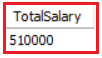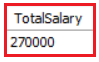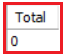# MySQL SUM Function

## MySQL SUM Function with Examples

In this article, I am going to discuss the MySQL SUM Function with Examples. Please read our previous article where we discussed COUNT Function in MySQL with Examples.

##### MySQL SUM Function:

The MySQL SUM Function returns the total sum of a given numeric column. The SUM function will only work on numeric data types. For columns containing string values, it will always return 0. Following is the syntax to use the SUM Function in MySQL.

SELECT SUM(column) FROM TableName;

We are going to use the following Employee table to understand the MySQL SUM function with Examples.Please use the following SQL Script to create and populate the Employee table with the required sample data.

```CREATE DATABASE Company;
USE Company;

CREATE TABLE Employee (
Id INT PRIMARY KEY,
Name VARCHAR(45) NOT NULL,
Department VARCHAR(45) NOT NULL,
Salary FLOAT NOT NULL,
Gender VARCHAR(45) NOT NULL,
Age INT NOT NULL,
City VARCHAR(45) NOT NULL
);

INSERT INTO Employee VALUES (1001, 'John Doe', 'IT', 35000, 'Male', 25, 'London');
INSERT INTO Employee VALUES (1002, 'Mary Smith', 'HR', 45000, 'Female', 27, 'London');
INSERT INTO Employee VALUES (1003, 'James Brown', 'Finance', 50000, 'Male', 28, 'London');
INSERT INTO Employee VALUES (1004, 'Mike Walker', 'Finance', 50000, 'Male', 28, 'London');
INSERT INTO Employee VALUES (1005, 'Linda Jones', 'HR', 75000, 'Female', 26, 'London');
INSERT INTO Employee VALUES (1006, 'Anurag Mohanty', 'IT', 35000, 'Male', 25, 'Mumbai');
INSERT INTO Employee VALUES (1007, 'Priyanla Dewangan', 'HR', 45000, 'Female', 27, 'Mumbai');
INSERT INTO Employee VALUES (1008, 'Sambit Mohanty', 'IT', 50000, 'Male', 28, 'Mumbai');
INSERT INTO Employee VALUES (1009, 'Pranaya Kumar', 'IT', 50000, 'Male', 28, 'Mumbai');
INSERT INTO Employee VALUES (1010, 'Hina Sharma', 'HR', 75000, 'Female', 26, 'Mumbai');
```
##### Example: SUM Function with Numeric Data Type

In our Employee table, the Id, Salary, and Age columns have numeric data types. Let us calculate the Total Salary of all employees. To do so, use the SUM function and pass Salary as a parameter to the SUM Function as shown in the below SQL Query.

SELECT SUM(Salary) As TotalSalary FROM Employee;

Now when you execute the above query, you will get the following output.##### Example: SUM Function with Group by Clause in MySQL

Now we will calculate the Total Salary of each department. To do so, we need to pass the Salary column to the SUM function as well as we need to group the record by department using the GROUP BY clause as shown in the below SQL Query.

```SELECT Department, SUM(Salary) As TotalSalary
FROM Employee
GROUP BY Department;```

Now when you execute the above SQL query, you will get the following output.##### Example: SUM Function with WHERE Clause in MySQL

Now we need to calculate the total salary of all Male Employees present in the Employee table. To do so, we need to pass the Salary column as a parameter to the SUM function and filter the records by using the WHERE clause as shown in the below SQL Query.

```SELECT SUM(Salary) As TotalSalary
FROM Employee
WHERE Gender = 'Male';```

Now when you execute the above SQL query, you will get the following output.##### Example: SUM with String Values

If we pass the string column value to the SUM function, then we will get 0 as the result. In the below query, we are passing the Name column to the SUM function.

SELECT SUM(Name) As Total FROM Employee;

Now when you execute the above SQL query, you will get the following output.